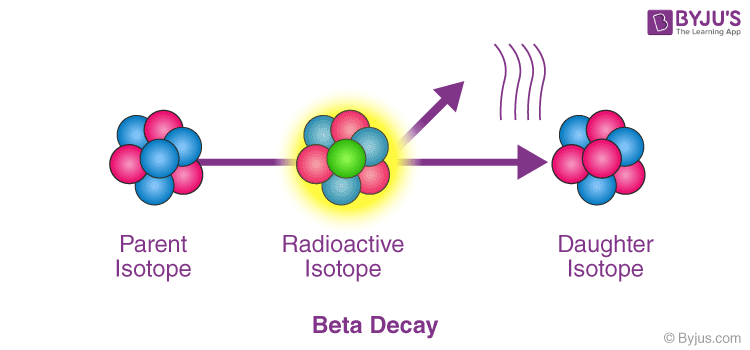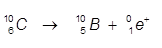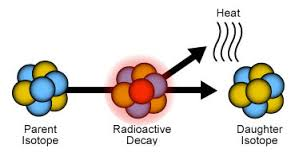# Beta Decay

Radioactivity refers to the particles which are emitted from nuclei as a result of nuclear instability. The spontaneous decay or breakdown of an atomic nucleus is known as Radioactive Decay. This decay in a nucleus causes the release of energy and matter from the nucleus.

The 3 most common forms of Radioactive decay are:

1. Alpha Decay (Helium nucleus is emitted)
2. Beta Decay (Electrons are emitted)
3. Gamma Decay (High energy photons are emitted)

## What happens in Beta Decay?

Beta-decay is a type of radioactive decay in which a beta ray is emitted from an atomic nucleus. During beta decay, the proton in the nucleus is transformed into a neutron and vice versa. If a proton is converted to neutron, it is known as β+ decay. Similarly, if a neutron is converted to a proton, it is known as β- decay. Due to the change in the nucleus, a beta particle is emitted. The beta particle is a high-speed electron when it is a β- decay and a positron when it is a β+ decay.

### Beta Decay Example

One of the examples of beta decay is the β- decay of carbon atom.Here, a neutron of carbon is converted into a proton and the emitted beta particle is an electron.

Similarly, the β+ decay of carbon-10 can be represented by an equation as follows:β+ decay of carbon-10

Here, the proton of the carbon atom is converted into a neutron and the emitted beta particle is a positron.

## Types of Beta Decay

There are three different Beta Decay types:

1. Electron emission
2. Electron capture
3. Positron emission

### Electron Emission

The process of ejection or emission of electron from the nucleus is known as electron emission. After the emission, the charge of the nucleus increases by one.

### Electron Capture

Electron capture is the phenomenon where the nuclei decay by capturing one of the electrons that surround the nucleus. This leads to a decrease of one in charge of the nucleus.

### Positron Emission

It is the third form of beta decay. A positron is an antimatter equivalent of an electron & has the same mass as of an electron, but bares the opposite charge of an electron. Positron decay produces a daughter nuclide with one less positive charge on the nucleus than the parent.Positron Emission

## Fermi’s Theory of Beta Decay

Fermi’s theory of beta decay or Fermi’s interaction is an illustration of beta decay by Enrico Fermi in 1933.  Enrico Fermi created the world’s first nuclear reactor.

He proposed that four fermions directly interacting with one another, at one vertex. This interaction explains beta decay of a neutron by direct coupling of a neutron with an electron, a neutrino (later determined to be an antineutrino), and a proton.